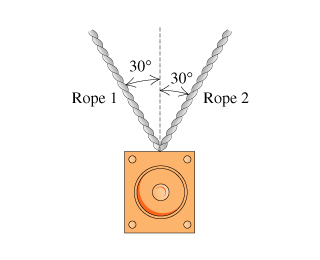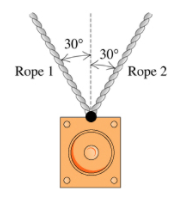# Problem: A 20-kg loudspeaker is suspended 2.0 m below the ceiling by two ropes that are each 30° from vertical. What is the tension in the ropes?Identify the forces acting on the loudspeaker and then draw them on the free-body diagram. Draw the vectors starting from the black dot. The location and orientation of the vectors will be graded. The length of the vectors will not be graded.

###### FREE Expert Solution

To solve this problem, we need to first know the forces acting on the loudspeaker.

We also need to use Newton's second law:

$\overline{){\mathbf{\Sigma }}{\mathbf{F}}{\mathbf{=}}{\mathbf{m}}{\mathbf{a}}}$

We have tension acting on rope 1, T1.

Tension acting on rope 2, T2.

The weight of the loudspeaker, mg.

85% (323 ratings)###### Problem Details

A 20-kg loudspeaker is suspended 2.0 m below the ceiling by two ropes that are each 30° from vertical. What is the tension in the ropes?Identify the forces acting on the loudspeaker and then draw them on the free-body diagram. Draw the vectors starting from the black dot. The location and orientation of the vectors will be graded. The length of the vectors will not be graded.